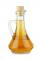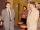# Solution

What amount of 80% solution and what amount of water is needed to mix in order to obtain two liters of 60% solution of this mixture?

Result

a =  1.5
b =  0.5

#### Solution:

a+b=2
0.8 a = 0.60 (a+b)

a+b = 2
0.2a-0.6b = 0

a = 32 = 1.5
b = 12 = 0.5

Calculated by our linear equations calculator.

Leave us a comment of example and its solution (i.e. if it is still somewhat unclear...):

Showing 0 comments:Be the first to comment!#### To solve this example are needed these knowledge from mathematics:

Our percentage calculator will help you quickly calculate various typical tasks with percentages. Do you have a linear equation or system of equations and looking for its solution? Or do you have quadratic equation? Tip: Our volume units converter will help you with converion of volume units.

## Next similar examples:

1. PharmacyAt the pharmacy are in one container 20% solution in the second 50% solution of disinfectant. They need to prepare 4 L of 48-percent solution. What amount of solution from each container is needed to mix?
2. CompetitorsIn the first round of slalom fell 15% of all competitors and in the second round another 10 racers. Together, 40% of all competitors fell. What was the total number of competitors?
3. Fifth of the numberThe fifth of the number is by 24 less than that number. What is the number?
4. ConcentrationHow 0.5 liters of 8% vinegar diluted to a concentration of 20 hundredths % of vinegar? How many liters of water must be pour?
5. BookAlena read a book at speed 15 pages per day. If she read twice as fast she should read a book four days earlier. How many pages have a book?
6. Rabbits 3Viju has 40 chickens and rabbits. If in all there are 90 legs. How many rabbits are there with Viju?
7. ATC campThe owner of the campsite offers 79 places in 22 cabins. How many of them are triple and quadruple?
8. Boxes200 boxes have been straightened in three rows. The first was 13 more than in the second, and in the second was one fifth more than in the third one. How many boxes are in each row?
9. Factory and divisionsThe factory consists of three auxiliary divisions total 2,406 employees. The second division has 76 employees less than 1st division and 3rd division has 212 employees more than the 2nd. How many employees has each division?
10. ClassroomThere are eighty more girls in the class than boys. Boys are 40 percent and girls are 60 percent. How many are boys and how many girls?
11. RavensOn two trees sitting 17 ravens. If 3 ravens flew from first to second tree and 5 ravens took off from second tree then the first tree has 2 times more ravens than second tree. How many ravens was originally on every tree?
12. PearsAndrew, Lenka and Rasto have together 232 pears. Lenka has 28 more than Rasto and Rasto pears have 96 more than Andrew. Determine how much each of them has pears.
13. GirlsThe children's competition was attended by 63 girls, which is 30% of all children's participants. How many children attended this competition?
14. Two workersTwo workers make 138 parts/items. The first one produces 30% more than the second. How many items will each produce?
15. Equations - simpleSolve system of linear equations: x-2y=6 3x+2y=4
16. BlackberriesDaniel, Jolana and Stano collected together 34 blackberries. Daniel collected 8 blackberries more than Jolana, Jolana 4 more than Stano. Determine the number blackberries each collected .
17. PercentsHow many percents is 900 greater than the number 750?